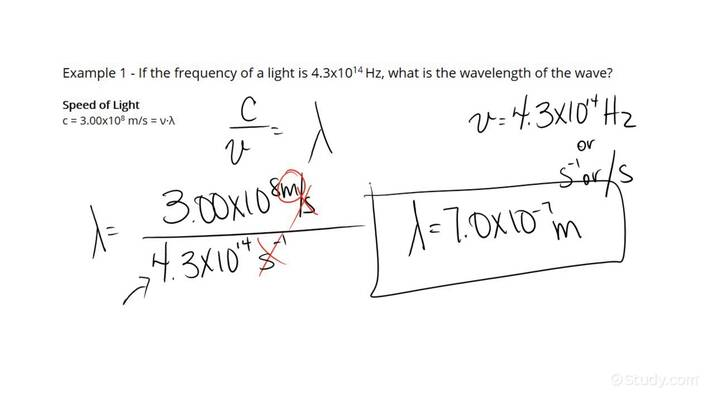# How to Find the Wavelength of Light Using its FrequencyAn error occurred trying to load this video.

Try refreshing the page, or contact customer support.

• 00:11 How to Find the…
• 02:54 How to Find the…
APRIL MANGANO, Kirsten Wordeman
• Instructors
APRIL MANGANO

April has been tutoring students, elementary to college level, in varying subjects for over 10 years. April has a Bachelor of Physics from Rutgers University and is currently working toward a Master's of Applied Physics from John's Hopkins University.

•
Kirsten Wordeman

Kirsten has taught high school biology, chemistry, physics, and genetics/biotechnology for three years. She has a Bachelor's in Biochemistry from The University of Mount Union and a Master's in Biochemistry from The Ohio State University. She holds teaching certificates in biology and chemistry.

## How to Find the Wavelength of Light Using its Frequency

When finding the wavelength of light using its frequency, follow these easy steps:

Step 2: Take the information provided within the problem and plug it into the wavelength equation to determine the wavelength .

## What is a Wave?

A wave can be expressed as an oscillation carrying energy from one point to another through space and time.

## What is a Wavelength?

A wavelength is defined as the distance between two successive wave peaks, the highest points of the wave, or two successive troughs, the lowest points of the wave.

## What is Frequency?

Wave frequency describes the number of waves passing through a fixed point over a specific amount of time.

## How to Find the Wavelength of Light Using its Frequency

When given the frequency of a wave, one can use the following formula to determine the wavelength:

{eq}\lambda = \frac{v}{f} {/eq}

Where {eq}\lambda {/eq} is the wavelength which is measured in meters, v is the velocity of the wave measured in meters per second (m/s), and f is the frequency of the wave which is measured in Hertz (Hz).

This formula can be used when two out of the three variables are given

However, if only one variable is provide, you can use the following formula:

{eq}\lambda = \frac{c}{f} {/eq}

Where {eq}\lambda {/eq} is the wavelength which is measured in meters, c is the speed of light which equals {eq}3 \times 10^{8} {/eq} m/s, and f is frequency which is measured in Hertz (Hz).

## Examples of Determining Wavelength from Frequency

The following examples will help illustrate how to determine wavelength from frequency.

### Example 1

If the frequency of light is {eq}4.7 \times 10^{8} {/eq} Hz, what will its wavelength be?

After reading the problem, we can see that only one variable is given. Therefore, we know that we can use the formula

{eq}\lambda = \frac{c}{f} {/eq}

Step 2: Take the information provided within the problem and plug it into the wavelength equation to determine the wavelength

Now we can plug the values we have into the equation and solve for lambda which is wavelength.

{eq}\lambda = \frac{3 \times 10^{8} m/s }{4.7 \times 10^{8} Hz} = 0.64 m {/eq}

### Example 2

What will the length of the light wave be, if the frequency is {eq}2.4 \times 10^{8} {/eq} Hz and its velocity is indicated to be {eq}2.6 \times 10^{8} {/eq} meters per second?

After reading the problem, we can see that two variables are given. Therefore, we know that we can use the formula

{eq}\lambda = \frac{v}{f} {/eq}

Step 2: Take the information provided within the problem and plug it into the wavelength equation to determine the wavelength

Now we can plug the values we have into the equation and solve for lambda which is wavelength.

{eq}\lambda = \frac{2.6 \times 10^{8} m/s }{2.4 \times 10^{8} Hz} = 1.08 m {/eq}